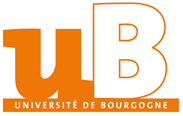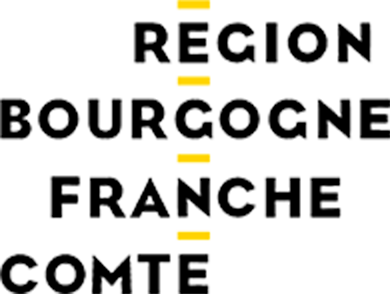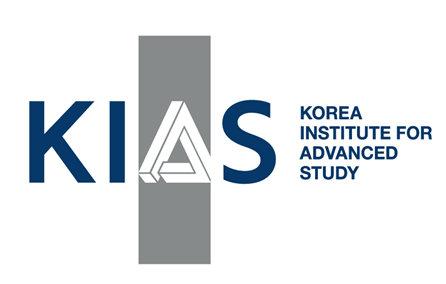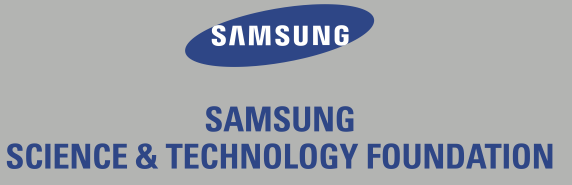# Low dimensional actions of 3-manifold groups

## Dijon (France), 4-8 November 2019

Description: Group actions on manifolds constitute a very active subject nowadays, which lies at the interface between geometry, dynamical systems and geometric group theory. Actions of fundamental groups of closed surfaces are by far the most studied objects, because of the deep relationship with hyperbolic geometry and more generally Teichmüller theory. Although of similar geometric nature, actions of fundamental groups of hyperbolic 3-manifolds have not received much attentions. From a geometric and group-theoretical point of view, these groups have been a very central object in the last decade, so the time seems appropriate to investigate more their low dimensional dynamics. The aim of this workshop is to gather international experts of the fields to describe the state of the art, and to try settling the basis for a more general theory.

Here is the schedule

Mini-courses: Lectures notes compiled by Hang Lu Su: see her blog.

• Harry Baik (Korea Advanced Institute of Science & Technology)
Flows and foliations in 3-manifolds and laminar groups
Abstract: Thurston developed the theory of universal circles which connects the theory of codimension-one foliations in 3-manifold and 3-manifolds group actions on the circle. This motivated work of many authors including Calegari, Dunfield, Fenley, Gabai, Kazez, Roberts. We will review the outline of the construction of universal circle when 3-manifold admits a nice foliation or flow, and motivates the theory of laminar groups from the perspective of the universal circle. We will also mention some of the recent developments in this direction.
• Steve Boyer (Université du Québec, Montréal)
The orderability 3-manifold groups and topology
Abstract: Many decades of work culminating in Perelman's proof of Thurston's geometrisation conjecture have shown that a closed, connected, orientable, prime 3-dimensional manifold $$W$$ is essentially determined by its fundamental group $$\pi_1(W)$$. Consequently, an important problem in low-dimensional topology is to describe the basic properties of $$W$$ in terms of $$\pi_1(W)$$. These lectures will provide the background for a discussion of the conjectural picture which has emerged concerning the properties of $$W$$ characterised by the existence of a left-invariant total order (i.e. a left-order) on $$\pi_1(W)$$. The first lecture will survey that part of the theory of ordered groups relevant to our study. In the second we will show that $$\pi_1(W)$$ admits a left-order if and only if it acts non-trivially on the real line by orientation-preserving homeomorphisms and use this criterion to determine precisely which Seifert fibre spaces have left-orderable fundamental groups. In the final lecture we will examine the orderability of the fundamental groups of various families of hyperbolic manifolds. This will lead to a discussion of the L-space conjecture, which posits the equivalence between the following three conditions on $$W$$:
1. the existence of a left-order on $$\pi_1(W)$$;
2. the existence of a co-oriented taut foliation of $$W$$;
3. the condition that $$W$$ not be a Heegaard-Floer L-space.
• Antonin Guilloux (Sorbonne Université)
An introduction to arithmetic lattices of $$\mathrm{PSL}(2,\mathbb{C})$$
Abstract: We will look at a special class of lattices of $$\mathrm{PSL}(2,\mathbb{C})$$ called arithmetic lattices. and hereby of hyperbolic 3-manifolds. After a general presentation of their definition and properties, we will give hints on what is so special about this lattices including considerations on the volume of those manifolds, the richness of their covers, or the significance of being able to change the base field.
• Luisa Paoluzzi (Université d'Aix-Marseille)
An introduction to (hyperbolic) 3-manifolds and their fundamental groups
Abstract: It follows from Thurston's geometrization conjecture, now proved by Perelman, that one can reduce the study of closed 3-manifolds to that of geometric 3-manifolds, and in particular of hyperbolic ones. Indeed, every closed 3-manifold can be decomposed in a unique way into geometric pieces. Of course, such decomposition induces a decomposition of the fundamental group of the manifold via Seifert-Van Kampen theorem. After a quick survey of the eight 3-dimensional Thurston geometries, I will discuss the Kneser-Milnor decomposition of manifolds along spheres and the Jaco-Shalen-Johannson one along tori, as well as their group-theoretical counterparts. Finally, I will point out the paramount importance of the study of (closed and punctured) surface groups and their automorphisms in the understanding of 3-manifold groups, as a consequence of the virtual fibering conjecture of Thurston proved by Agol and Wise.

Research talks:

• Thierry Barbot (Université d'Avignon):
Orbital equivalence classes of Anosov flows on circle bundles over surfaces
Abstract: in 1981, E. Ghys proved that any Anosov flow on a circle bundle over a surface $$S$$ is a finite covering of the geodesic flow of $$S$$. In this talk, I will present the proof of the following fact: if the index of the covering is odd, then all these flows on a given manifold are orbitally equivalent one to the other. If not, there are two orbital equivalence classes. This is a joint work with S. Fenley.
• Michel Boileau (Université d'Aix-Marseille)
Pseudo-Anosov surfaces in 3-manifolds
Abstract: An embedded surface $$F$$ in a closed orientable irreducible 3-manifold is said a pseudo-Anosov surface if $$F$$ is preserved by a homeomorphism of $$M$$ which induces a pseudo-Anosov map on $$F$$. Motivated by questions in partially hyperbolic dynamic F. Rodriguez Hertz, J. Rodriguez Hertz and R. Ures have shown that an Anosov torus in a closed orientable irreducible 3-dimensional manifold is always the fiber of a bundle over $$S^1$$. This cannot be always the case for a pseudo-Anosov surface of higher genus, and it is unknown which three manifolds admit a pseudo-Anosov surface. The goal of this talk is to discuss some properties of pseudo-Anosov surfaces. This is a work in progress with Pierre Derbez and Shicheng Wang.
• Yan Mary He (University of Toronto and Université du Luxembourg):
Identities for hyperconvex Anosov representations
Abstract: Basmajian's identity expresses the volume of the boundary of a compact hyperbolic manifold as a sum over the orthogeodesics on the manifold. In this talk, I will introduce identities for real hyperconvex Anosov representations (in the sense of Pozzetti-Sambarino-Wienhard) and show how to extend them to the complex ones in the $$\mathrm{PGL}(n,\mathbb{C})$$-character variety which requires understanding the action of the surface group on the projective space.
• Alan Reid (Rice University)
Is the free group profinitely rigid?
Abstract: A f.g. residually finite group $$G$$ is called profinitely rigid, if whenever $$H$$ is a f.g. residually finite group with isomorphic profinite completion to that of $$G$$, then $$H$$ is isomorphic to $$G$$. It remains open as to whether a free group of rank at least 2 is profinitely rigid. This talk will discuss recent progress on this question, as well as discussing what properties, a potential non-free example with profinite completion a free profinite group, would have.

Organizers:

Practical information: The workshop will take place at the Institut de Mathématiques de Bourgogne of Université of Bourgogne (Dijon, France). We can offer accommodation for most participants that register, giving priority to the youngest.

Supports:
Université de Bourgogne
Institut de Mathématiques de Bourgogne (UMR CNRS 5584)
Région Bourgogne-Franche-Comté